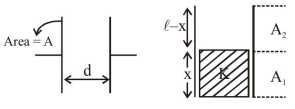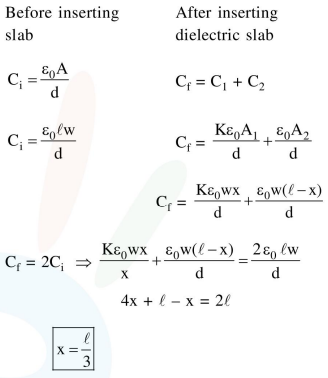# A parallel plate capacitor has plate of length

Question:

A parallel plate capacitor has plate of length ' $l$ ', width 'w' and separation of plates is 'd'. It is connected to a battery of emf V. A dielectric slab of the same thickness 'd' and of dielectric constant $\mathrm{k}=4$ is being inserted between the plates of the capacitor. At what length of the slab inside plates, will be energy stored in the capacitor be two times the initial energy stored?

1. $l / 4$

2. $l / 2$

3. $l / 3$

4.  $2 l / 3$

Correct Option: 3,

Solution: